Bohr combined classical and early quantum concepts and gave his theory in the form of three postulates. These are:

1. Bohr’s first postulate was that an electron in an atom could revolve in certain stable orbits without the emission of radiant energy, contrary to the predictions of electromagnetic theory.
According to this postulate, each atom has certain definite stable states in which it can exist, and each possible state has definite total energy. These are called the stationary states of the atom.
2. Bohr’s second postulate defines these stable orbits. This postulate states that the electron revolves around the nucleus only in those orbits for which the angular momentum is some integral multiple of h/2π where h is the Planck’s constant (= 6.6 × 10-34 J s). Thus the angular momentum (L) of the orbiting electron is quantized.
That is Ln = nh/2π
3. Bohr’s third postulate incorporated into atomic theory the early quantum concepts that had been developed by Planck and Einstein. It states that an electron might make a transition from one of its specified non-radiating orbits to another of lower energy. When it does so, a photon is emitted having energy equal to the energy difference between the initial and final states. The frequency of the emitted photon is then given by

hv = Ei – Ef

where Ei and Ef are the energies of the initial and final states and Ei > Ef.

### De Broglie’s hypothesis

that electrons have a wavelength λ = h/mv gave an explanation for Bohr’s quantized orbits by bringing in the wave-particle duality. The orbits correspond to circular standing waves in which the circumference of the orbit equals a whole number of wavelengths.

Bohr’s model is applicable only to hydrogenic (single electron) atoms. It cannot be extended to even two electron atoms such as helium. This model is also unable to explain for the relative intensities of the frequencies emitted even by hydrogenic atoms.

### Raddi of Bohr’s Stationary Orbits: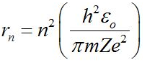Form the above equation; we can see that r ∝ n2 (here Z is atomic number). It means radii of stationary orbits are in the ratio 12 : 22 : 33….. and so on. So, stationary orbits are not equally spaced.

### Velocity of Electron in Bohr’s Stationary Orbits:

The velocity is given by,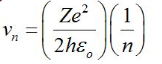This means v∝ (1/n).

### Total energy of Electron in Bohr’s Stationary Orbits:

Total energy is given by,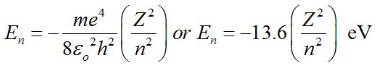The negative sign of the total energy of an electron moving in an orbit means that the electron is bound with the nucleus. Energy will thus be required to remove the electron from the hydrogen atom to a distance infinitely far away from its nucleus (or proton in hydrogen atom).

Note: Bohr model is valid for only one- electron atoms/ions; an energy value, assigned to each orbit, depends on the principal quantum number n in this model. We know that energy associated with a stationary state of an electron depends on n only, for one- electron atoms/ions. For a multi-electron atom/ion, this is not valid.

### Energy levels of Hydrogen Atom:

The total energy of an electron in nth orbit of a hydrogen atom is given by En = (-13.6/n2) eV.

As a result of the quantization condition of angular momentum, the electron orbits the nucleus at only specific radii. For a hydrogen atom it is given by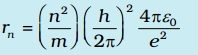The total energy is also quantized: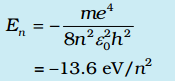The n = 1 state is called ground state. In hydrogen atom the ground state energy is –13.6 eV. Higher values of n correspond to excited states (n > 1). Atoms are excited to these higher states by collisions with other atoms or electrons or by absorption of a photon of right frequency.

Putting n = 1, 2, 3… is above equation, we get the energies of electron as, E1 = -13.6 eV , E2 = -3.4 eV , E3 = -1.51 eV…

These energy levels of hydrogen atom are represented in energy level diagram given below.